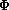Electron. J. Diff. Eqns., Vol. 2004(2004), No. 68, pp. 1-12.

### Positive solutions for the-Laplacian whenis a sup - multiplicative - like function George L. Karakostas

Abstract:
We provide sufficient conditions for the existence of positive solutions of a boundary-value problem for a one dimensional-Laplacian ordinary differential equation with deviating arguments, whereis a sup-multiplicative-like function (in a sense introduced here) and the boundary conditions include nonlinear expressions at the end points. For this end, we use the Krasnoselskii fixed point theorem in a cone. The results obtained improve and generalize known results in  and elsewhere.

Submitted February 5, 2004. Published May 4, 2004.
Math Subject Classifications: 34B15, 34B18.
Key Words: Boundary value problems, positive solutions, Krasnoselskii's fixed point theorem.

Show me the PDF file (233K), TEX file, and other files for this article.George L. Karakostas Department of Mathematics University of Ioannina, 451 10 Ioannina, Greece email: gkarako@cc.uoi.gr